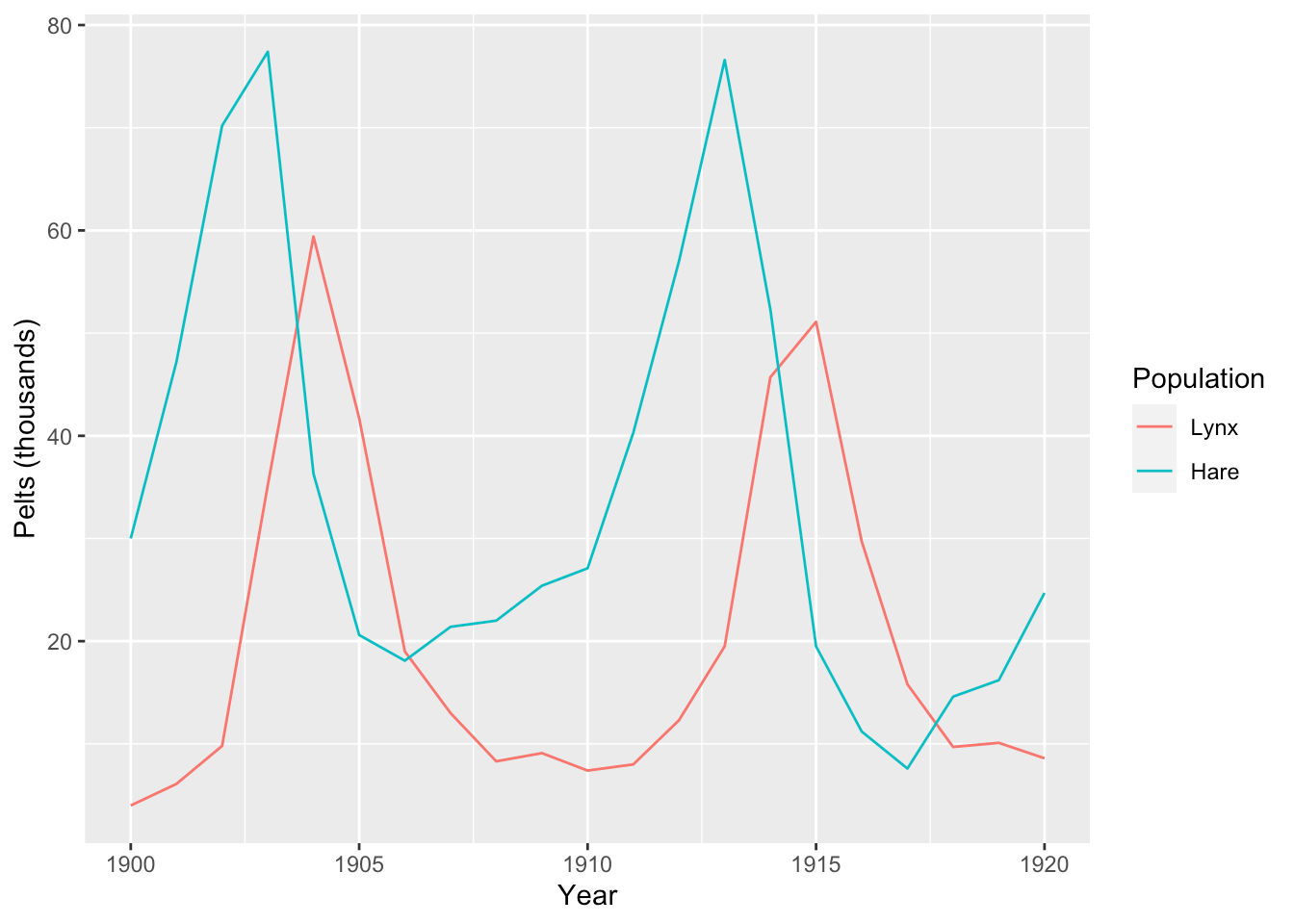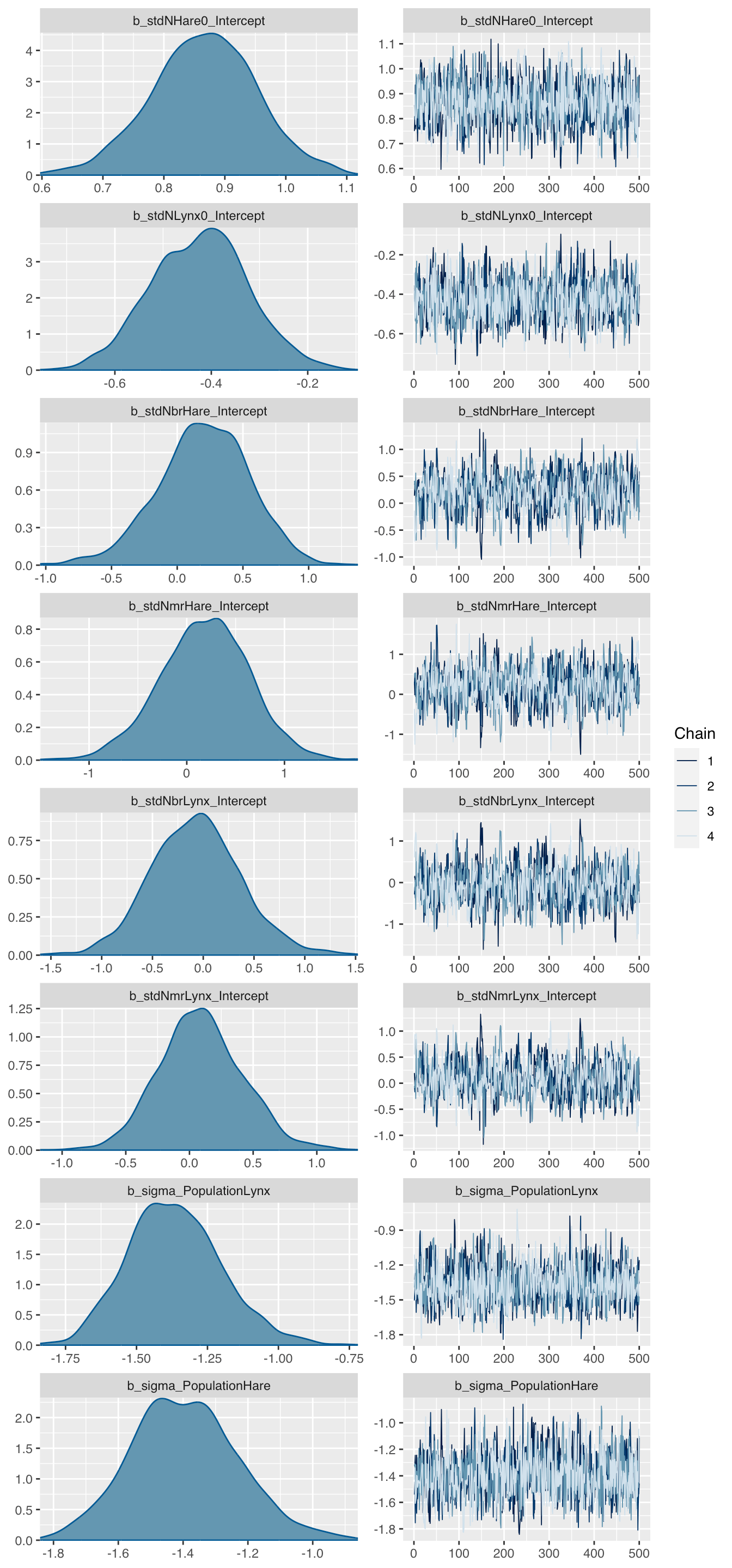Want to share your content on R-bloggers? click here if you have a blog, or here if you don't.

This article illustrates how ordinary differential equations and multivariate observations can be modelled and fitted with the brms package () in R1.

As an example I will use the well known Lotka-Volterra model (, ) that describes the predator-prey behaviour of lynxes and hares. Bob Carpenter published a detailed tutorial to implement and analyse this model in Stan and so did Richard McElreath in Statistical Rethinking 2nd Edition ().

Here I will use brms as an interface to Stan. With brms I can write the model using formulas similar to glm or lmer directly in R, avoiding to code up the model in Stan. Having said that, I will have to write a little bit of Stan for the ODEs and pass them on via the brm function.

# Data

The data to model shows the number of pelts in thousands of Canadian hares and lynxes from 1900 – 1920:

library(data.table)
Lynx_Hare <- data.table(
Year = 1900:1920,
Lynx = c(4, 6.1, 9.8, 35.2, 59.4, 41.7, 19, 13, 8.3, 9.1, 7.4, 8,
12.3, 19.5, 45.7, 51.1, 29.7, 15.8, 9.7, 10.1, 8.6),
Hare = c(30, 47.2, 70.2, 77.4, 36.3, 20.6, 18.1, 21.4, 22, 25.4,
27.1, 40.3, 57, 76.6, 52.3, 19.5, 11.2, 7.6, 14.6, 16.2, 24.7))
##    Year Lynx Hare
## 1: 1900  4.0 30.0
## 2: 1901  6.1 47.2
## 3: 1902  9.8 70.2
## 4: 1903 35.2 77.4
## 5: 1904 59.4 36.3
## 6: 1905 41.7 20.6# Model

The classic Lotka-Volterra model is a system of two autonomous ordinary differential equations (ODEs), describing the interaction between hares and lynxes. The four ODE parameters related to the birth and mortality rates of the two species. Finally, there are two further parameters for the initial states at time $$t=0$$:

\begin{aligned} \frac{dH}{dt} & = (b_H - m_H L ) H \\ \frac{dL}{dt} & = (b_L H - m_L) L \\ H(0) & = H_0 \\ L(0) & = L_0 \\ \end{aligned}

Note, the data only shows the number of pelts, i.e. the number of trapped and killed animals not the actual population statistics.

Assuming no population can become extinct and all parameters have to be positive, I will assume log-normal distributions for the process and the birth and mortality parameters.

# Implementation in brms

library(brms)
library(cmdstanr)
library(parallel)
nCores <- detectCores()

## Data preperations

The data was presented as a table with two columns, showing the time series for the hares and lynxes separately - a multivariate time series.

However, in order to model this data with brms the data has to be transformed into a univariate time series. The trick is to introduce indicator variables for the long format of the data. In addition I add a new time variable, which starts at 1, rather than 1900, since the ODEs in Stan expect an initial state at $$t=0$$.

LH <- melt(data.table(Lynx_Hare), id.vars = "Year",
measure.vars = c("Lynx", "Hare"),
variable.name = "Specie",
value.name = "Pelts")
LH[, := (
delta = ifelse(Specie %in% "Lynx", 1, 0),
Specie = factor(Specie),
t = Year - min(Year) + 1)]
##    Year Specie Pelts delta t
## 1: 1900   Lynx   4.0     1 1
## 2: 1901   Lynx   6.1     1 2
## 3: 1902   Lynx   9.8     1 3
## 4: 1903   Lynx  35.2     1 4
## 5: 1904   Lynx  59.4     1 5
## 6: 1905   Lynx  41.7     1 6

## ODE model in Stan

The implementation of the ODEs in Stan is very straightforward, but note below the integration step and the use of the indicator variable delta from my data set and how I use it to select the relevant metric from the multivariate output of the integrated ODEs. For delta = 0 the LV function returns the hare component only and for delta = 1 the lynx component.

LotkaVolterra <- "
// Sepcify dynamical system (ODEs)
real[] ode_LV(real t, real [] y,  real [] theta,
real [] x_r, int[] x_i){
real dydt;

dydt = (theta - theta * y ) * y; // Hare
dydt = (theta * y - theta) * y; // Lynx

return dydt;
}
// Integrate ODEs and prepare output
real LV(real t, real Hare0, real Lynx0,
real brHare, real mrHare,
real brLynx, real mrLynx,
real delta){
real y0;     // Initial values
real theta;  // Parameters
real y[1, 2];   // ODE solution
// Set initial values
y0 = Hare0; y0 = Lynx0;
// Set parameters
theta = brHare; theta = mrHare;
theta = brLynx; theta = mrLynx;
// Solve ODEs
y = integrate_ode_rk45(ode_LV,
y0, 0, rep_array(t, 1), theta,
rep_array(0.0, 0), rep_array(1, 1),
0.001, 0.001, 100); // tolerances, steps
// Return relevant specie values
return (y[1,1] * (1 - delta) +
y[1,2] * delta);
}
"

## Formula

To write the model formula in brms I make use of the nlf function, short for non-linear function, not only to map the median behaviour of the process, but also to transform standardised Normal(0, 1) priors to log-normal priors. In addition, I allow for different process variances for the two species.

frml <-  bf(
Pelts ~ eta,
nlf(eta ~ log(
LV(t, Hare0, Lynx0,
brHare, mrHare,brLynx, mrLynx, delta)
)
),
nlf(Hare0 ~ 10 * exp(stdNHare0)),
nlf(Lynx0 ~ 10 * exp(stdNLynx0)),
nlf(brHare ~ 0.5 * exp(0.25 * stdNbrHare)),
nlf(mrHare ~ 0.025 * exp(0.25 * stdNmrHare)),
nlf(brLynx ~ 0.025 * exp(0.25 * stdNbrLynx)),
nlf(mrLynx ~ 0.8 * exp(0.25 * stdNmrLynx)),
stdNHare0 ~ 1,  stdNLynx0 ~ 1,
stdNbrHare ~ 1, stdNmrHare ~ 1,
stdNbrLynx ~ 1, stdNmrLynx ~ 1,
sigma ~ 0 + Specie,
nl = TRUE)

## Priors

Where possible I try to stick to standardised Normal priors and use nlf to transform those to what I think are sensible ranges for the model.

mypriors <- c(
prior(normal(0, 1), nlpar = "stdNHare0"),
prior(normal(0, 1), nlpar = "stdNLynx0"),
prior(normal(0, 1), nlpar = "stdNbrHare"),
prior(normal(0, 1), nlpar = "stdNmrHare"),
prior(normal(0, 1), nlpar = "stdNbrLynx"),
prior(normal(0, 1), nlpar = "stdNmrLynx"),
prior(normal(-1, 0.5), class = "b",
coef= "SpecieHare",  dpar= "sigma"),
prior(normal(-1, 0.5), class = "b",
coef= "SpecieLynx",  dpar= "sigma")
)

## Model run

With the preparations done, I can start running the model with brm. I use cmdstan as the backend and feed the ODE model using the stanvars argument.

mod <- brm(
frml, prior = mypriors,
stanvars = stanvar(scode = LotkaVolterra, block = "functions"),
data = LH, backend = "cmdstan",
family = brmsfamily("lognormal", link_sigma = "log"),
seed = 1234, iter = 1000,
chains = 4, cores = nCores,
file = "LotkaVolterraCMDStan.rds")

I should add that I tried the rstan backend, but to no avail. I received the following error statements: lognormal_lpdf: Location parameter is nan, but must be finite!, integrate_ode_rk45: initial state is inf, but must be finite!. I can’t get my head around why they occurred, apart from the fact that rstan uses Stan 2.12, while cmdstan uses Stan 2.24.

# Model output

The model run takes about 10 minutes on my 2015 MacBook Air. The output looks promising:

mod
##  Family: lognormal
##   Links: mu = identity; sigma = log
## Formula: Pelts ~ eta
##          eta ~ log(LV(t, Hare0, Lynx0, brHare, mrHare, brLynx, mrLynx, delta))
##          Hare0 ~ 10 * exp(stdNHare0)
##          Lynx0 ~ 10 * exp(stdNLynx0)
##          brHare ~ 0.5 * exp(0.25 * stdNbrHare)
##          mrHare ~ 0.025 * exp(0.25 * stdNmrHare)
##          brLynx ~ 0.025 * exp(0.25 * stdNbrLynx)
##          mrLynx ~ 0.8 * exp(0.25 * stdNmrLynx)
##          stdNHare0 ~ 1
##          stdNLynx0 ~ 1
##          stdNbrHare ~ 1
##          stdNmrHare ~ 1
##          stdNbrLynx ~ 1
##          stdNmrLynx ~ 1
##          sigma ~ 0 + Specie
##    Data: LH (Number of observations: 42)
## Samples: 4 chains, each with iter = 1000; warmup = 500; thin = 1;
##          total post-warmup samples = 2000
##
## Population-Level Effects:
##                      Estimate Est.Error l-95% CI u-95% CI Rhat Bulk_ESS
## stdNHare0_Intercept      0.86      0.09     0.67     1.03 1.01     1500
## stdNLynx0_Intercept     -0.42      0.10    -0.62    -0.21 1.00     1136
## stdNbrHare_Intercept     0.21      0.34    -0.45     0.84 1.00      535
## stdNmrHare_Intercept     0.19      0.45    -0.71     1.05 1.00      618
## stdNbrLynx_Intercept    -0.07      0.43    -0.89     0.78 1.00      667
## stdNmrLynx_Intercept     0.09      0.33    -0.55     0.70 1.01      550
## sigma_SpecieLynx        -1.37      0.16    -1.66    -1.05 1.00     1505
## sigma_SpecieHare        -1.39      0.16    -1.68    -1.06 1.00     1619
##                      Tail_ESS
## stdNHare0_Intercept      1378
## stdNLynx0_Intercept      1030
## stdNbrHare_Intercept      910
## stdNmrHare_Intercept      982
## stdNbrLynx_Intercept     1031
## stdNmrLynx_Intercept      856
## sigma_SpecieLynx         1278
## sigma_SpecieHare         1282
##
## Samples were drawn using sample(hmc). For each parameter, Bulk_ESS
## and Tail_ESS are effective sample size measures, and Rhat is the potential
## scale reduction factor on split chains (at convergence, Rhat = 1).

Let’s take a look at the parameter distribution plot:

theme_update(text = element_text(family = "sans"))
plot(mod, N=8)The plots look all sensible, but the parameters don’t look familiar, so let’s transform them back to the original scale. Mind you, these will be estimates of the medians not means, as I use log-normal distributions:

rbind(
Hare0 = 10*exp(fixef(mod)[c('stdNHare0_Intercept'),]),
Lynx0 = 10*exp(fixef(mod)[c('stdNLynx0_Intercept'),]),
brHare = 0.5*exp(0.25*fixef(mod)[c('stdNbrHare_Intercept'),]),
mrHare = 0.025*exp(0.25*fixef(mod)[c('stdNmrHare_Intercept'),]),
brLynx = 0.025*exp(0.25*fixef(mod)[c('stdNbrLynx_Intercept'),]),
mrLynx = 0.8*exp(0.25*fixef(mod)[c('stdNmrLynx_Intercept'),]),
SigmaHare = exp(fixef(mod)[c('sigma_SpecieHare'),]),
SigmaLynx = exp(fixef(mod)[c('sigma_SpecieLynx'),])
)
##              Estimate   Est.Error        Q2.5       Q97.5
## Hare0     23.61742242 10.93711654 19.58839635 28.03852179
## Lynx0      6.59691147 11.06972203  5.40357863  8.06813008
## brHare     0.52734106  0.54433143  0.44675493  0.61658220
## mrHare     0.02622329  0.02799179  0.02091373  0.03251019
## brLynx     0.02455565  0.02784820  0.02000280  0.03035897
## mrLynx     0.81738699  0.86813662  0.69798412  0.95404341
## SigmaHare  0.24949470  1.17075951  0.18674121  0.34526648
## SigmaLynx  0.25476458  1.17204758  0.18921634  0.35152437

## Plot posterior simulations

Before I can draw simulations from the posterior predictive distributions, I have to expose the Stan functions to R. Unfortunately, at the moment this only works with brms using rstan as a backend. Hence, I recompile the model with rstan so that I can expose the functions to R.

library(rstan)
modRstan <- brm(
frml, prior = mypriors,
stanvars = stanvar(scode = LotkaVolterra, block = "functions"),
data = LH, backend = "rstan", chains = 0,
family = brmsfamily("lognormal", link_sigma = "log"),
file = "LotkaVolterraRStan")
expose_functions(modRstan, vectorize = TRUE, cacheDir = "~/Downloads/")

Finally, I can run simulations from the posterior predictive model and compare the simulated output with the data.

library(tidybayes)
pred <- predicted_draws(mod, newdata = LH, n= 1000)
ggplot(pred, aes(x = Year, y = Pelts)) +
stat_lineribbon(aes(y = .prediction),
.width = c(.99, .95, .8, .5),
color = "#08519C") +
geom_point(data = pred) + labs(y="Pelts (thousands)") +
scale_fill_brewer() + facet_wrap(~ Specie)This plot suggests again that this model is a not unreasonable.

# Summary

Although the model seems to describe the data well, it has its limitations:

• The solutions of the ODEs for a fixed set of parameters
are defined by the initial values, i.e. there are only stable orbits
• No specie can become extinct, i.e if the initial value was greater 0
• There are many other factors impacting the birth and death rates of hares and lynxes apart from the interaction between the two species

However, this example demonstrated how multivariate ODE models can be fitted with brms. For more complex examples of multivariate ODEs with brms see the case studies in section 5 of ().

# Session Info

session_info <- (sessionInfo()[-8])
utils:::print.sessionInfo(session_info, local=FALSE)
## R version 4.0.3 (2020-10-10)
## Platform: x86_64-apple-darwin17.0 (64-bit)
## Running under: macOS Big Sur 10.16
##
## Matrix products: default
## BLAS:   /Library/Frameworks/R.framework/Versions/4.0/Resources/lib/libRblas.dylib
## LAPACK: /Library/Frameworks/R.framework/Versions/4.0/Resources/lib/libRlapack.dylib
##
## attached base packages:
##  parallel  stats     graphics  grDevices utils     datasets  methods
##  base
##
## other attached packages:
##  ggplot2_3.3.3        data.table_1.13.6